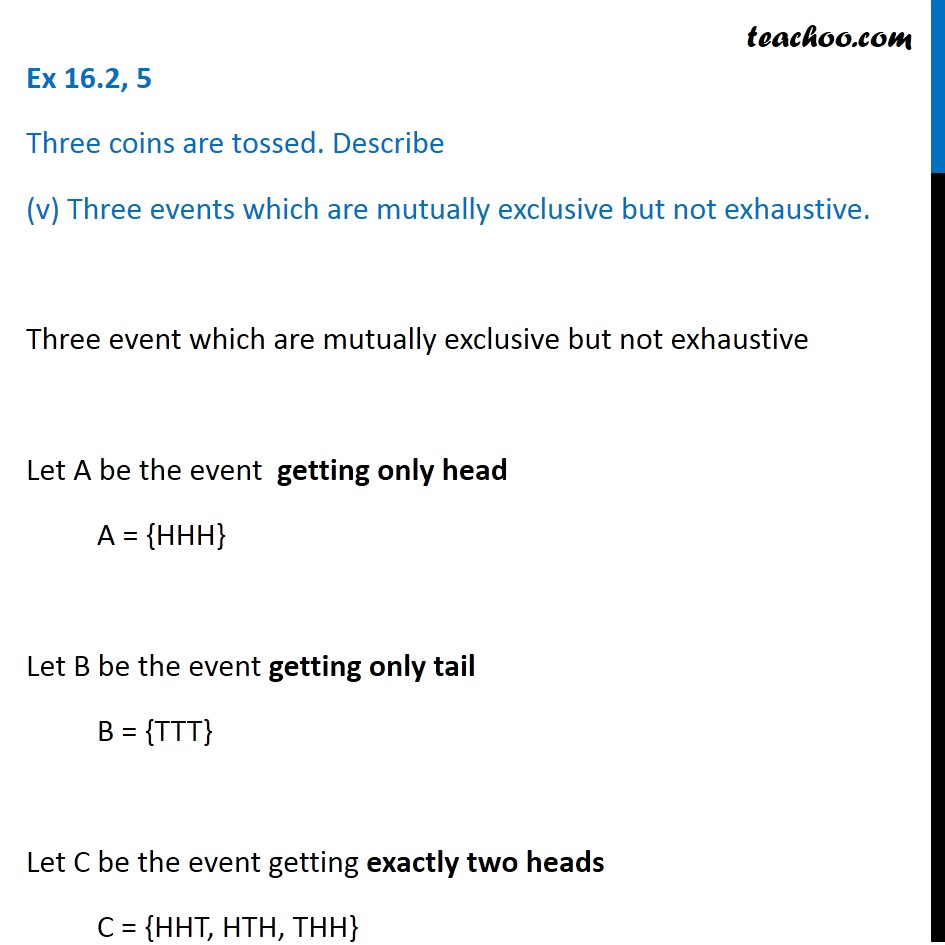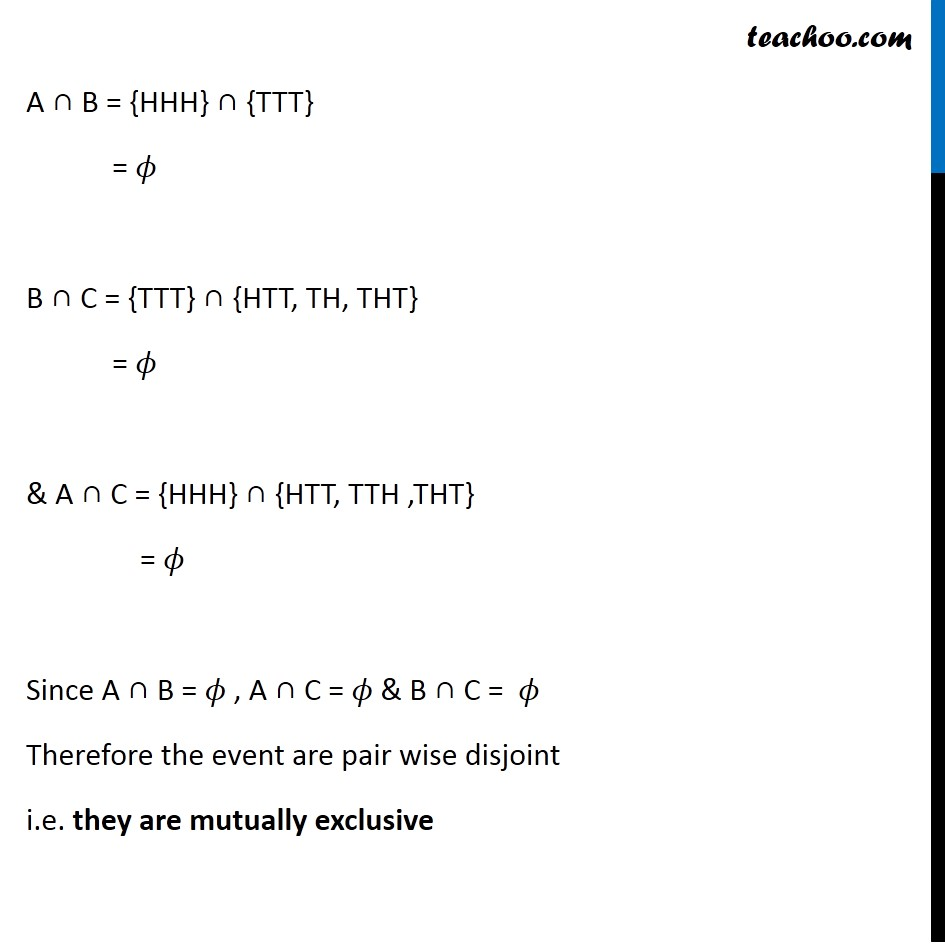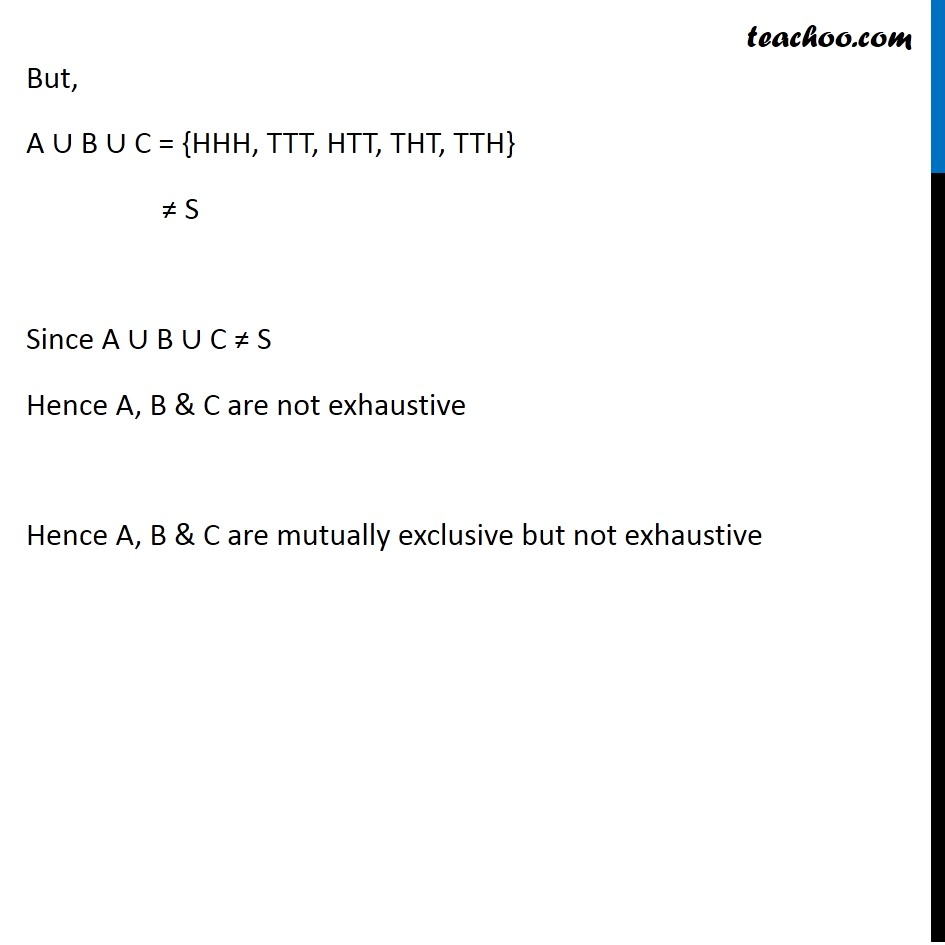Ex 14.1

Chapter 14 Class 11 Probability
Serial order wiseLearn in your speed, with individual attention - Teachoo Maths 1-on-1 Class

### Transcript

Ex 14.1, 5 Three coins are tossed. Describe (v) Three events which are mutually exclusive but not exhaustive. Three event which are mutually exclusive but not exhaustive Let A be the event getting only head A = {HHH} Let B be the event getting only tail B = {TTT} Let C be the event getting exactly two heads C = {HHT, HTH, THH} A ∩ B = {HHH} ∩ {TTT} = 𝜙 B ∩ C = {TTT} ∩ {HTT, TH, THT} = 𝜙 & A ∩ C = {HHH} ∩ {HTT, TTH ,THT} = 𝜙 Since A ∩ B = 𝜙 , A ∩ C = 𝜙 & B ∩ C = 𝜙 Therefore the event are pair wise disjoint i.e. they are mutually exclusive But, A ∪ B ∪ C = {HHH, TTT, HTT, THT, TTH} ≠ S Since A ∪ B ∪ C ≠ S Hence A, B & C are not exhaustive Hence A, B & C are mutually exclusive but not exhaustive